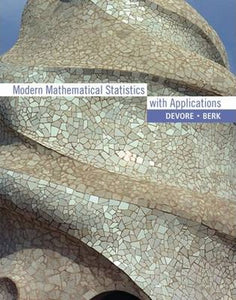# Modern Mathematical Statistics with Application by Kenneth Berk

• R 950.00
Unit price per
Tax included.

ISBN: 9780534404734

Cengage Learning Inc. 05 Feb 2006

Hard Cover, 848 pages

Many mathematical statistics texts are heavily oriented toward a rigorous mathematical development of probability and statistics, without emphasizing contemporary statistical practice. MODERN MATHEMATICAL STATISTICS WITH APPLICATIONS strikes a balance between mathematical foundations and statistical practice. Accomplished authors Jay Devore and Ken Berk first engage students with real-life problems and scenarios and then provide them with both foundational context and theory. This book follows the spirit of the Committee on the Undergraduate Program in Mathematics (CUPM) recommendation that every math student should study statistics and probability with an emphasis on data analysis.